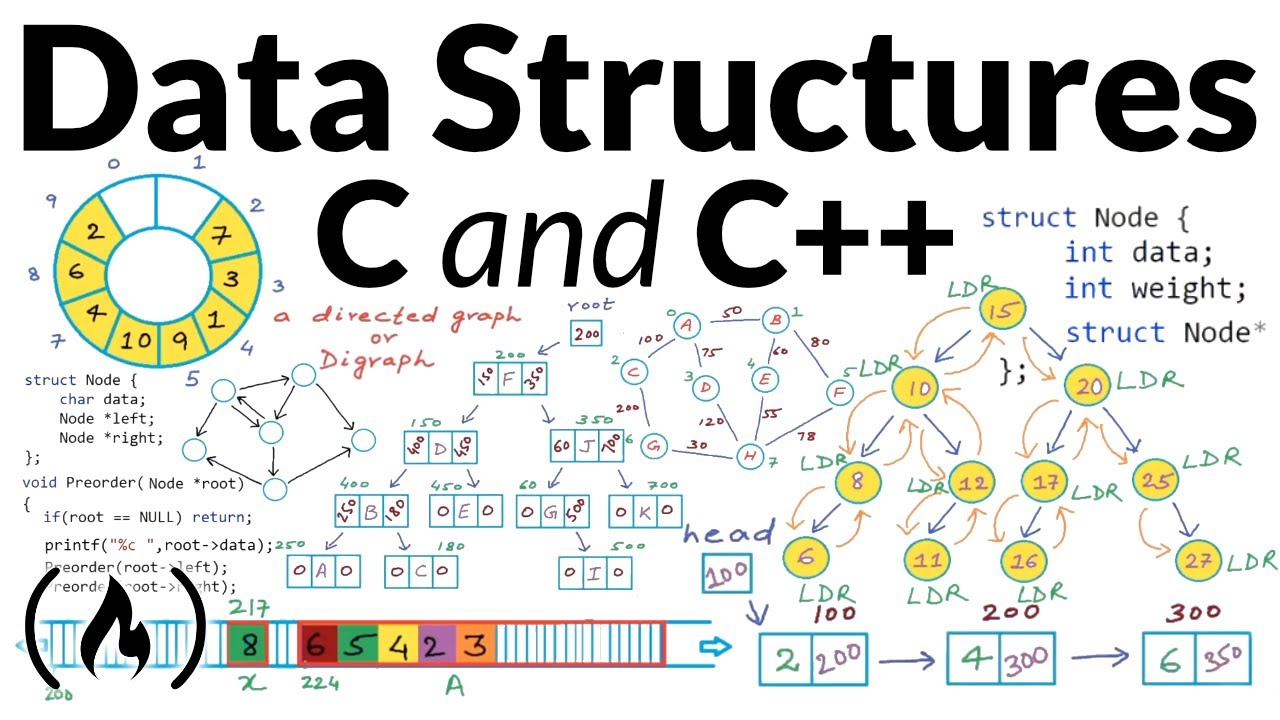# Data Structures - Full Course Using C and C++Learn about data structures in this comprehensive course. We will be implementing these data structures in C or C++.

Learn about data structures in this comprehensive course. We will be implementing these data structures in C or C++.

⭐️ Course Contents ⭐️
⌨️ (0:00:00) Introduction to data structures
⌨️ (0:06:33) Data Structures: List as abstract data type
⌨️ (0:19:40) Introduction to linked list
⌨️ (0:36:50) Arrays vs Linked Lists
⌨️ (0:49:05) Linked List - Implementation in C/C++
⌨️ (1:03:02) Linked List in C/C++ - Inserting a node at beginning
⌨️ (1:15:50) Linked List in C/C++ - Insert a node at nth position
⌨️ (1:31:04) Linked List in C/C++ - Delete a node at nth position
⌨️ (1:43:32) Reverse a linked list - Iterative method
⌨️ (1:57:21) Print elements of a linked list in forward and reverse order using recursion
⌨️ (2:11:43) Reverse a linked list using recursion
⌨️ (2:20:38) Introduction to Doubly Linked List
⌨️ (2:27:50) Doubly Linked List - Implementation in C/C++
⌨️ (2:43:09) Introduction to stack
⌨️ (2:51:34) Array implementation of stacks
⌨️ (3:04:42) Linked List implementation of stacks
⌨️ (3:15:39) Reverse a string or linked list using stack.
⌨️ (3:32:03) Check for balanced parentheses using stack
⌨️ (3:46:14) Infix, Prefix and Postfix
⌨️ (3:59:14) Evaluation of Prefix and Postfix expressions using stack
⌨️ (4:14:00) Infix to Postfix using stack
⌨️ (4:32:17) Introduction to Queues
⌨️ (4:41:35) Array implementation of Queue
⌨️ (4:56:33) Linked List implementation of Queue
⌨️ (5:10:48) Introduction to Trees
⌨️ (5:26:37) Binary Tree
⌨️ (5:42:51) Binary Search Tree
⌨️ (6:02:17) Binary search tree - Implementation in C/C++
⌨️ (6:20:52) BST implementation - memory allocation in stack and heap
⌨️ (6:33:55) Find min and max element in a binary search tree
⌨️ (6:39:41) Find height of a binary tree
⌨️ (6:46:50) Binary tree traversal - breadth-first and depth-first strategies
⌨️ (6:58:43) Binary tree: Level Order Traversal
⌨️ (7:10:05) Binary tree traversal: Preorder, Inorder, Postorder
⌨️ (7:24:33) Check if a binary tree is binary search tree or not
⌨️ (7:41:01) Delete a node from Binary Search Tree
⌨️ (7:59:27) Inorder Successor in a binary search tree
⌨️ (8:17:23) Introduction to graphs
⌨️ (8:34:05) Properties of Graphs
⌨️ (8:49:19) Graph Representation part 01 - Edge List
⌨️ (9:03:03) Graph Representation part 02 - Adjacency Matrix
⌨️ (9:17:46) Graph Representation part 03 - Adjacency List